# React 高阶组件其实很简单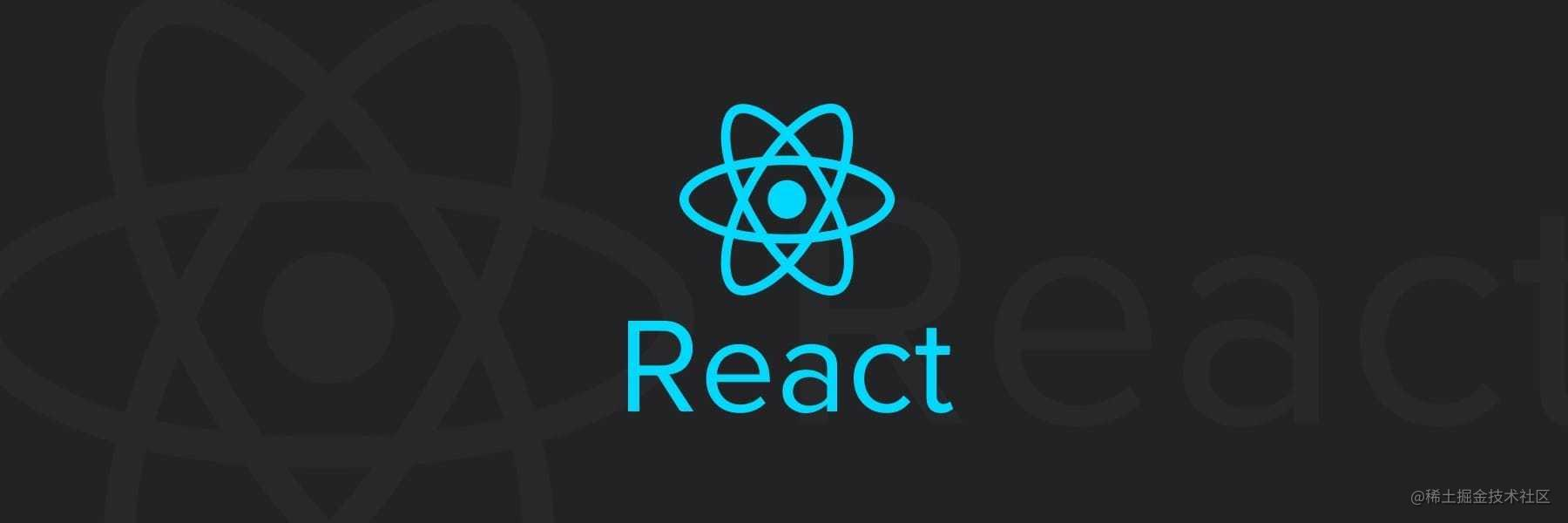## 什么是高阶组件

``````const EnhancedComponent = highOrderComponent(WrappedComponent);

``````import React, { Component } from 'react';

export default (WrappedComponent) => {
return class EnhancedComponent extends Component {
// do something
render() {
return <WrappedComponent />;
}
}
}

## 使用高阶组件

React 中 受控组件，是通过 `state` 来控制用户的输入行为，所以我们需要通过监听 `onChange` 来更新 `state`，例如：

``````import React, { Component } from 'react';

class Input extends Component {
constructor(props) {
super(props);

this.state = {
value: '',
}
}

handleChange = (e) => {
this.setState({
value: e.target.value,
});
}

render() {
return (
<input type="text" value={this.state.value} onChange={this.handleChange} />
);
}
}

export default Input;

``````import React, { Component } from 'react';

export default (WrappedComponent) => {
return class InputHOC extends Component {
constructor(props) {
super(props);
this.state = {
value: '',
}
}

handleChange = (e) => {
this.setState({
value: e.target.value,
});
}

render() {
const passProps = {
value: this.state.value,
onChange: this.handleChange,
}
return <WrappedComponent {...passProps} />;
}
}
}

``````import React, { Component } from 'react';
import InputHOC from './InputHOC';

import './style.css';

class Input extends Component {
render() {
return (
<input className="input" type="text" {...this.props} />
)
}
}

export default InputHOC(Input);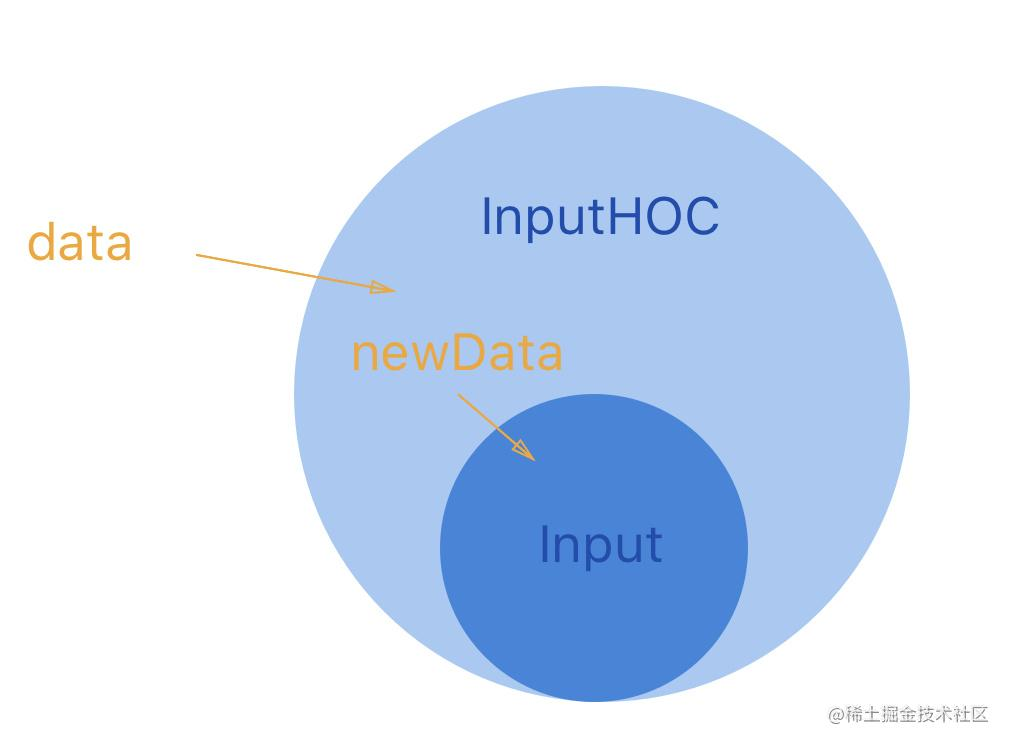## 一些注意点

### 参数

``````export default (WrappedComponent, data) => {
return class EnhancedComponent extends Component {
// do something with data
render() {
return <WrappedComponent />;
}
}
}

### 命名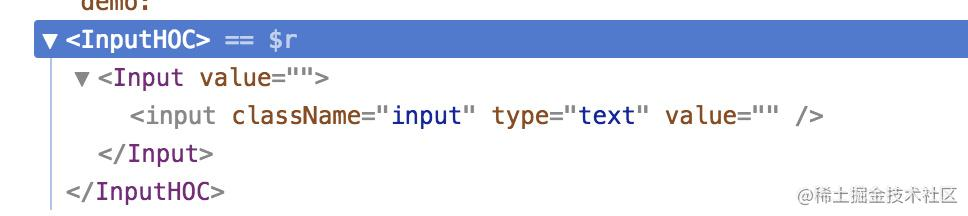``````import React, { Component } from 'react';

const getDisplayName = (WrappedComponent) => {
return WrappedComponent.displayName ||
WrappedComponent.name ||
'Component'
}

export default (WrappedComponent, validate) => {
return class InputHOC extends Component {
static displayName = `InputHOC(\${getDisplayName(WrappedComponent)})`;
constructor(props) {
super(props);
this.state = {
value: '',
}
}

handleChange = (e) => {
console.log(e);
this.setState({
value: e.target.value,
});
}

render() {
const passProps = {
value: this.state.value,
onChange: this.handleChange,
}
return <WrappedComponent {...passProps} />;
}
}
}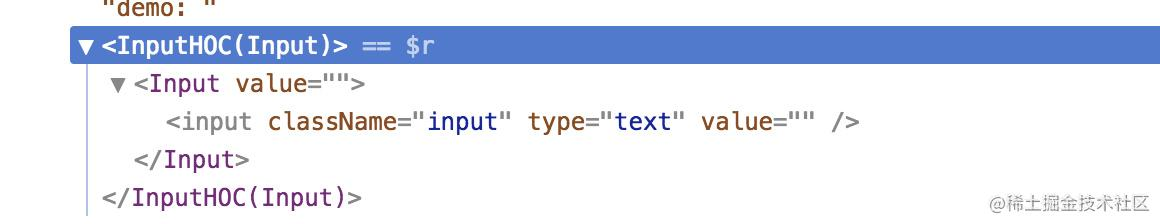### 多层高阶组件

``````Input = InputHOC(Input);
Input = InputHHOC(Input);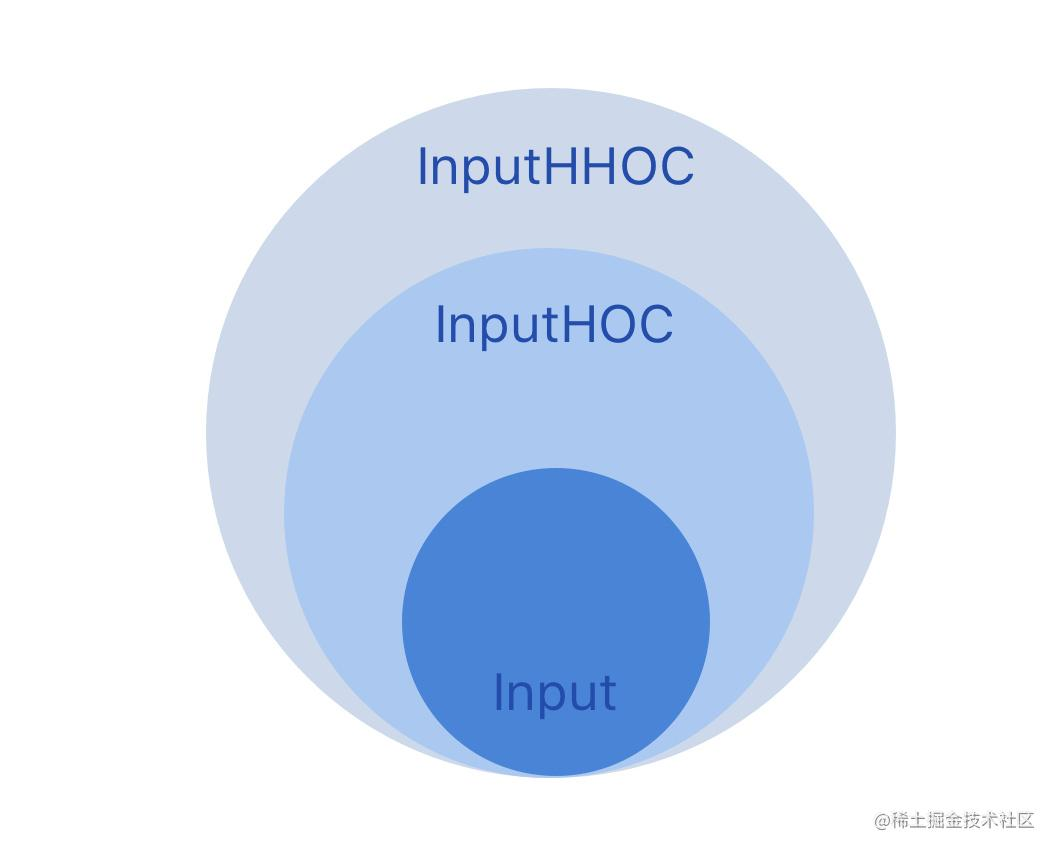## 总结

• 高阶组件是一个函数，而不是组件
• 组件是把 props 转化成 UI，高阶组件是把一个组件转化成另一个组件
• 高阶组件的作用是复用代码
• 高阶组件对应设计模式里的装饰者模式